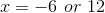# High School Math : Graphing Circle Functions

## Example Questions

### Example Question #1 : Circle Functions

Find the-intercepts for the circle given by the equation: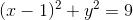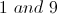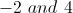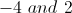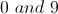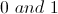Explanation:

To find the-intercepts (where the graph crosses the-axis), we must set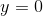. This gives us the equation: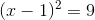Because the left side of the equation is squared, it will always give us a positive answer. Thus if we want to take the root of both sides, we must account for this by setting up two scenarios, one where the value inside of the parentheses is positive and one where it is negative. This gives us the equations: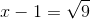and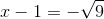We can then solve these two equations to obtain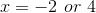.

### Example Question #1 : Circle Functions

Find the-intercepts for the circle given by the equation: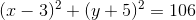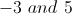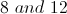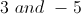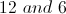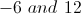Explanation:

To find the-intercepts (where the graph crosses the-axis), we must set. This gives us the equation: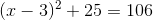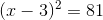Because the left side of the equation is squared, it will always give us a positive answer. Thus if we want to take the root of both sides, we must account for this by setting up two scenarios, one where the value inside of the parentheses is positive and one where it is negative. This gives us the equations: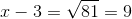and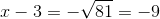We can then solve these two equations to obtain